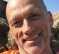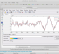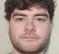## Nyquist frequency & odd even FFTStarted by 2 years ago17 replieslatest reply 2 years ago1265 views

Hi All,

I came to realise something odd about Nyquist frequency (a bit late though).

When I generate it, it comes out only real. So I expected it to mirror around dc. But it doesn't, it only settles at negative Nyquist bin.

After some research the explanation given by some is that it depends on FFT being odd or even.

I tried both even and odd FFT and true with odd fft the tone is seen on both +/-ve Nyquist.

However, the way I see why odd FFT will do that is just that it smears the tone as it contain half cycle while the even case contains full cycles.

In other words proper FFT should be even but then how do we explain that such tone being real yet living in -ve Nyquist only. Something is not right here.

I don't have any practical issue here but just wanted to clear my thoughts.

Any contributions welcomed.

[ - ]Hello Kaz,

The DFT works with an even or odd number of points.... Every sampled data sinewave has a continuous periodic frequency response. The DFT simply samples this continuous periodic spectrum. Traditionally at multiples of 2 pi/N where N is the number of input samples, which can be even or odd.... if you want the spectral samples to coincide with the center frequency of the sinewave then the sinewave must have an integer number of cycles in the input interval span. when you have an odd number of samples the interval spans zero to N-1 + 1/2 ... the extension from the last sample to the next sample which is sample zero again as you complete the trip around the circle. When you have an even number of samples the last interval from sample N-1 to zero is a full interval, not a half interval... we are unwrapping a circle and displaying it on a line what matlab can do is FFTshift which puts index zero in the array center and starts the spectral sampling at -N/2 and stops at +(N/2)-1... with an odd number of samples there is no spectral sample at +/- N/2.

se attached paper

[ - ]Many thanks fred,

Let us keep it within the context of my use of Matlab fft as a reliable processing function.

I got a stream of even number data points of +1/-1

I apply fft and look at tone it is on -ve Nyquist.

x = exp(j*2*pi*1/2*(0:1000-1));

y = fft(x);

plot(abs(y),'*-');

Only bin 501 is seen occupied.

I am simply asking how come this real tone is not mirroring.

If I go for odd number of samples or append zeros I will be force smearing the bins and lose track of input power bin centre which I know is the Nyquist.

So let me limit the question to two elements:

i. Why this real tone is not mirroring? does the rule has this exception?.

ii. Should I use odd number of samples or append zeros to force smear the power? I don't think so.

[ - ]Hi Kaz,

There have been a number of responses here along the same lines so my apologies if I'm not adding much of anything new. First, the snippet of MATLAB code you provided is critical to streamlining and expediting the discussion. Thank you for that. Without that, the English language or any human language by itself clearly falls short in trying to convey the finer points of math and engineering.

I think the confusion is the conflation of the terms mirroring and smearing. These are two different things. And again, sorry, this is not intended to sound like a lecture. Mirroring is what happens when you have real tones and you look at their magnitude spectrum. You'll have mirror images about DC. Smearing is what happens when have signals of interest that are not periodic within an FFT frame. Those are very different things but they both lead to multiple frequency components in the FFT output.

>> i. Why this real tone is not mirroring? does the rule has this exception?.

Your signal of interest x is a complex tone (not a real tone), so by definition it should only have one non-zero value in the FFT (no mirroring)...as long as the signal lies on an FFT line. And by definition, and by careful construction, yours does. The FFT bin spacing is 1/1000 Hz. And you have a 0.5 Hz complex exponential. In MATLAB code, using your example:

Fs = 1; % Hz.

Nfft = 1000;

dF = Fs/Nfft;

fvec = [0:dF:Fs-dF]; % in Hz

freq_of_bin_501 = fvec(501) % which is 0.5 exact.

x = exp(j*2*pi*(1/2)*[0:Nfft-1]);

y = fft(x);

plot(fvec,abs(y),'*-');grid on

This is the perfect case. No need for any windowing, no spectral leakage issues to contend with, a single non-zero output in the fft magnitude.

>> Should I use odd number of samples or append zeros to force smear the power? I don't think so.

The short answer is no, not unless you want to demonstrate spectral leakage effects. All bets are off when your signal of interest is not periodic within an FFT frame. In this case, you get the smearing effect you mentioned.

You could do this for example:

Fs = 1; % Hz.

Nfft = 1001; % one bonus point

dF = Fs/Nfft;

fvec = [0:dF:Fs-dF]; % in Hz

x = exp(j*2*pi*(1/2)*[0:Nfft-1]); % same complex tone as before

y = fft(x);

plot(fvec,abs(y),'*-');grid on;

Now you get smearing where the smearing is mirrored with respect to the bin 501.5

fvec(501:502) = [0.4995   0.5005] % Hz

but that's a different form of mirroring as opposed to the symmetry we see with the fft magnitude of a real tone, e.g. x = sin(2*pi*(1/2)*[0:Nfft-1]);

Finally, as fharris mentioned, as a practical matter one never processes the FFT bins near Nyquist as these are not alias-free within some specified tolerance, say 80 dB, due to non-brickwall anti-alias filtering. That's another topic however. A typical percentage of the FFT bins kept would be 1/1.28 or the first 78.125% of the bins or in that ballpark.

[ - ]Thanks Kschutz,

Just to fix some issues you mentioned for sake of readers.

Using "exp" I actually expected real part of x as +1/-1. It should not have imaginary component as two samples per cycle cannot have 90 degree shift. I just used "exp" for convenience and you can select real part only.

I did not and never imply that mirroring is same as smearing.

Now I see what went wrong in my initial thoughts. An odd FFT will do the job as it covers ~-0.5 to ~+0.5 but I assumed its secondary effect is smearing that masks the observation.

I should have realised that smearing will be of low fraction of power but what odd FFT shows is indeed two peaks (mirroring + a bit of smearing).

Incidentally you used x = sin(2*pi*(1/2)*[0:Nfft-1]) at some point. It generates something weird sort of floating point noise. So I will avoid it. same happened with exp function so I suggest discarding imaginary part in this case.

It seems things at Nyquist are a bit weird.

[ - ]Hi Kaz,

I am 100% confident you know the difference between smearing and mirroring but I thought it worthwhile to clarify the terms/effects because your limiting use-case at Fs/2 sorts of brings the effects together into one magnitude spectrum. Typically they are independent effects. I'm referring to the odd, e.g. 1001 length, for the FFT use-case. In fact Fs/2 is the only signal frequency where the smearing just so happens to be mirrored, again, due to, as you said, the signal being only +1's and -1's. Most of us don't spend much time on signals at exactly Fs/2 as they typically are not of much signal processing value assuming they originated as part of a real-world analog process. Thus, your seemingly simple question is a bit of stumper and led to a longer than expected thread. Cheers!

[ - ]We can also see that dc itself behaves the same way. It is real and has one bin to go to. The +1/-1 tone seems to go to the -ve Nyquist bin only. I believe this is inherent in fft processing.

[ - ]Hi,

X value is complex exponential, x = exp(j*2*pi*1/2*(0:1000-1)); it will have a single frequency peak in the frequency domain.

Even for a real x, if x is at the highest frequency (0.5 : pi, +-1 values), mirrored value in the frequency domain is again at -pi, which is same

[ - ]+1/-1 alternating is real only.

It cannot have 90 degrees imaginary member to pair with because it is just two samples per cycle.

You can try fft(real(x)) if in doubt.

What I am saying there is no mirroring it is just one bin at -Nyquist.

[ - ]Yes, that mirroring is the same frequency for the Nyquist frequency (think about this way, +pi and -pi are the same, just like 0 and 2*pi are the same frequencies)

[ - ]I can think anything but my question is why fft is doing that when I know my frequency is real at Nyquist. Why it doesn't show it on +/-Nyquist?? or at +Nyquist at least??

[ - ]I think I got the answer.

It is inherent in fft bin definition. For example  1000 bin is spread as 500 negative bins, one dc and 499 positive bins so knowing that spacing is 1/1000 then only negative side can reach 0.5 (500/1000) while the positive side will see upto 499/1000

It is trivial weird issue of that odd dc bin but is worth it. Thanks all. being -ve or +ve here is meaningless as there is no imaginary element and can't be there with just two values per cycle. The fft simply swallowed the +ve 0.5

[ - ]incidentally, My version of the Nyquist Theorem is that the sample rate should exceed the two sided bandwidth.... if that is interpreted correctly the sineusoid can never be at the half sample rate and the odd part of the spectrum, which is also the imaginary part of the spectrum can't cancel. if the intent of the Nyquist criterion is to allow reconstruction of the continuous, band limited, signal from its sampled values... my interpretation is the valid one..... The question is by how much should the sample rate exceed the two sided bandwidth? the engineer's version of the Nyquist criterion is that fs should be equal to or greater than the two sided BW + transition BW of anti-aliasing filter... the transition BW is typically 10% to 20% of the two sided BW... CD player....2-sided BW = 40 kHz, fs=44.1 kHz, (4.1 kHz transition BW).... MP3 player 2 sided BW = 40 kHz, fs= 48 kHz, (8 kHz transition BW)

we always give reserve 10 to 20% of spectrum for filter transition BW... a 1-kHz spectrum analyzer (of a real signal) is often called a 400 line analyzer... positive frequencies.... 500 bins, displayed frequency 400 bins.... 20% transition BW.

fred

[ - ]If you have an even fft you will have one bin at the nyquist frequency.
The reason there is only 1 is because the +nyquist is identical to -nyquist.

The DFT correlates the input signal against exp(-j2πfn).
For the bin representing the Nyquist frequency f could be 0.5 or -0.5, they result in the same correlation.

exp(-j2π(0.5)n) == exp(-j2π(-0.5)n)

[ - ]That is how even FFT behaves for example for N = 1000:

left side bins: dc bin + 499 positive (but no +.5), total 500 bins

right side bins:500 bins (includes -0.5), total 500 bins

But odd FFT (1001) is different (symmetrical around dc bin):

left side: dc bin + 500 positive bins (includes ~+.5), total 501 bins

right side: 500 bins(includes ~-0.5), total 500

In other simple words: even FFT lies. odd FFT does not.

But odd FFT is hard to implement for sake of such possibly trivial "bug"

[ - ]The odd fft doesnt have a frequency bin @ the nyquist.

the frequency bins are determined by k/N if N is odd then k/N can never be equal to 0.5.

For N = 10, These are the frequencies that each output bin represents.
[-0.5 -0.4 -0.3 -0.2 -0.1  0.   0.1  0.2  0.3  0.4]
For N = 11, they are approx
[ 0, 0.09, 0.18, 0.27, 0.36, 0.45, 0.45, -0.36, -0.27, -0.18, -0.09]
note it does not reach 0.5fs the nyquist

[ - ]It could be but I am used to follow Matlab FFT and spacing:

even 1000 bins:

499 positive bins @ 1/1000 spacing = .4990

500 bins @1/1000 = 0.5 (negative side)

odd 1001 bins:

500 bins @ 1/1001 = 0.4995 either side

Do you agree with my view.

And not being 0.5 exact explains that bit of smearing for 0.5 tone

[ - ]